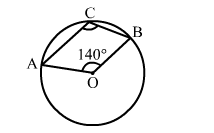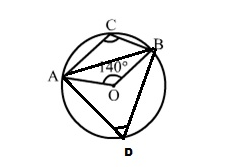# In the given figure, O is the centre of a circle and ∠AOB = 140°.

Question:

In the given figure, O is the centre of a circle and AOB = 140°. Then, ∠ACB = ?
(a) 70°

(b) 80°
(c) 110°
(d) 40°Solution:

(c) 110°
Join AB.
Then chord AB subtends ∠AOB at the centre and ∠ADB at a point D of the remaining parts of a circle.$\Rightarrow \angle \mathrm{ADB}=\frac{1}{2} \angle \mathrm{AOB}=\left(\frac{1}{2} \times 140^{\circ}\right)=70^{\circ}$